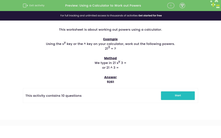# Using a Calculator to Work out Powers

In this worksheet, students work out powers using a calculator.Key stage:  KS 3

Curriculum topic:   Number

Curriculum subtopic:   Use Calculators/Technology for Accuracy

Difficulty level:#### Worksheet Overview

This worksheet is about working out powers using a calculator.

Example

Using the xy key or the ^ key on your calculator, work out the following powers.

213 = ?

Method

We type in 21 xy 3 =

or 21 ^ 3 =